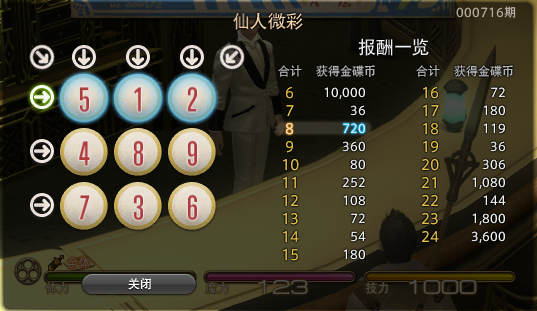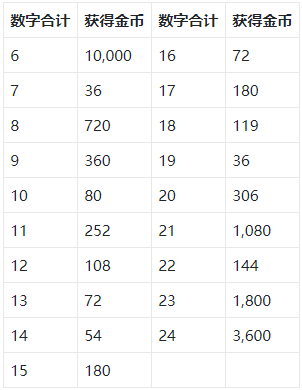# L1-8 刮刮彩票 (20分)（2020天梯赛）

“刮刮彩票”是一款网络游戏里面的一个小游戏。如图所示：输入样例:
1 2 3
4 5 6
7 8 0
1 1
2 2
2 3
7

1
5
6
180

#include<bits/stdc++.h>
using namespace std;
int book;

int main()
{
int a,sum=0,path,dx,dy,x,y,m=3;
for(int i=1;i<=3;i++)
{
for(int j=1;j<=3;j++)
{
cin>>a[i][j];
book[a[i][j]]++;
if(a[i][j]==0)
{
dx=i;
dy=j;
}
}
}
for(int i=1;i<=9;i++)
{
if(book[i]==0)
{
a[dx][dy]=i;
}
}
while(m--)
{
cin>>x>>y;
cout<<a[x][y]<<endl;
}
cin>>path;
if(path==1)
{
for(int i=1;i<=3;i++)
sum+=a[path][i];
}
if(path==2)
{
for(int i=1;i<=3;i++)
sum+=a[path][i];
}
if(path==3)
{
for(int i=1;i<=3;i++)
sum+=a[path][i];
}
if(path==4)
{
for(int i=1;i<=3;i++)
sum+=a[i];
}
if(path==5)
{
for(int i=1;i<=3;i++)
sum+=a[i];
}
if(path==6)
{
for(int i=1;i<=3;i++)
sum+=a[i];
}
if(path==7)
{
for(int i=1;i<=3;i++)
sum+=a[i][i];
}
if(path==8)
{
sum+=a+a+a;
}
if(sum==6)  cout<<"10000";
if(sum==7)  cout<<"36";
if(sum==8)  cout<<"720";
if(sum==9)  cout<<"360";
if(sum==10)  cout<<"80";
if(sum==11)  cout<<"252";
if(sum==12)  cout<<"108";
if(sum==13)  cout<<"72";
if(sum==14)  cout<<"54";
if(sum==15)  cout<<"180";
if(sum==16)  cout<<"72";
if(sum==17)  cout<<"180";
if(sum==18)  cout<<"119";
if(sum==19)  cout<<"36";
if(sum==20)  cout<<"306";
if(sum==21)  cout<<"1080";
if(sum==22)  cout<<"144";
if(sum==23)  cout<<"1800";
if(sum==24)  cout<<"3600";
return 0;
}


04-06226112-02225
11-291820
03-312864
01-201万+
12-02229
04-162115
11-282447
01-138445
08-0113万+
11-29480
11-291150
12-05296
08-18396
11-29473
12-10413
12-03198
12-13818